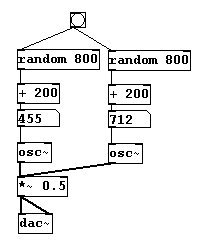## Appendix A. Solutions

Here you'll find the suggested solutions to the "More exercises" sections found at the end of every chapter. These are by no means comprehensible; in most cases, other correct solutions are possible.

## 2.2.1.2.8

a) Two simultaneous random melodies:b) Select (any) two different intervals using two bangs:c) Use "expr" to calculate exponential functions, e.g., y = x2 or y = x(2 + x) or y = 1 - (2x):